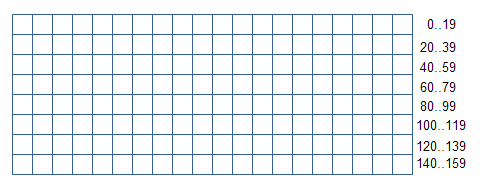# HTML5 Canvas: Pixel Manipulation

 Jakob Jenkov Last update: 2014-06-15

It is possible to get access to the individual pixels of an HTML5 canvas. You do so using an `ImageData` object. The `ImageData` object contains an array of pixels. By accessing this array you can manipulate the pixels. When you are done with the pixel manipulation, you need to copy the pixels onto a canvas to display them.

## Creating an ImageData Object

Creating an `ImageData` object is done using the 2D Context function `createImageData()`. Here is an example:

```var canvas  = document.getElementById("ex1");
var context = canvas.getContext("2d");

var width  = 100;
var height = 100;
var imageData = context.createImageData(width, height);
```

The `width` and `height` properties of the `createImageData()` function sets the width and height in pixels, of the pixel area represented by the `ImageData` object created. The example above creates an `ImageData` object with a 100 by 100 pixel area.

## ImageData Properties

The `ImageData` object has three properties:

• width
• height
• data

The `width` and `height` properties contain the width and height of the graphical data area.

The `data` property is a byte array containing the pixel values.

## Manipulating the Pixels

Each pixel in the `data` array consists of 4 bytes values. One value for the red color, green color and blue color, and a value for the alpha channel. The color of a pixel is determined by mixing red, green and blue together to make up the final color. The alpha channel tells how transparent the pixel is. Each of the red, green, blue and alph values can take values between 0 and 255.

Here is a code example that sets the color and alpha values of the first pixel:

```var pixelIndex = 0;
imageData.data[pixelIndex    ] = 255;  // red   color
imageData.data[pixelIndex + 1] =   0;  // green color
imageData.data[pixelIndex + 2] =   0;  // blue  color
imageData.data[pixelIndex + 3] = 255;
```

To read the values of a pixel, you write this code:

```var pixelIndex = 0;
var red   = imageData.data[pixelIndex    ];  // red   color
var green = imageData.data[pixelIndex + 1];  // green color
var blue  = imageData.data[pixelIndex + 2];  // blue  color
var alpha = imageData.data[pixelIndex + 3];
```

To access the values of subsequent pixels, increase the `pixelIndex` value by 4 (each pixel is made up of 4 array elements, as shown above).

You calculate the index of a given pixel like this:

``` var index = 4 * (x + y * width);
```

The `x` and `y` in the calculation is the x and y coordinate of the pixel to calculate the index of. Pixels in the `data` array are organized as one long sequence of pixels, starting with the top left pixel and moving vertically towards the right. When the end of the line is reached the pixel sequence continues from the leftmost pixel on the line below. Therefore, to calculate the index of a pixel located at x, y you need to multiply the y coordinate with the number of pixels per line, and add the x value to it.

Here is a diagram illustrating a 20 pixel wide, and 8 pixel high ImageData pixel array. In the right margin the indices of the pixels of each row is listed. As you can see, the enumeration of the indices start from 0 at the upper left corner, and increases towards the right. When the edge of a line is reached, the enumeration continues from the leftmost pixel of the line below, and continues towards the right.ImageData pixel grid - a 20 x 8 pixel grid. Pixels are indexed from the left upper corner towards the right, and line for line downwards.

## Copying Pixels Onto a Canvas

Once you have finished manipulating the pixels, you can copy them onto the canvas use the 2D Context function `putImageData()`. There are two versions of the `putImageFunction()`. The first version of the `putImageData()` function copies all the pixels onto the canvas. Here is an example:

```var canvasX = 25;
var canvasY = 25;

context.putImageData(imageData, canvasX, canvasY);
```

The `canvasX` and `canvasY` parameters are the x and y coordinate of where on the canvas to insert the pixels.

The second version of the `putImageData()` function can copy a rectangle of the pixels onto the canvas instead of all of the pixels. Here is a code example:

```var canvasX = 25;
var canvasY = 25;
var sx      = 0;
var sy      = 0;
var sWidth  = 25;
var sHeight = 25;

context.putImageData(imageData, canvasX, canvasY,
sx, sy, sWidth, sHeight);
```

The `sx` and `sy` parameters (sourceX and sourceY) are the x and y coordinates of the left upper corner of the rectangle to copy from the pixel array.

The `sWidth` and `sHeight` parameters (sourceWidth and sourceHeight) are the width and height of the rectangle to copy from the pixel array.

## Grabbing Pixels From a Canvas

It is also possible to grab a rectangle of pixels from a canvas into an `ImageData` object. This is done using the `getImageData()` function. Here is an example:

```var x      =  25;
var y      =  25;
var width  = 100;
var height = 100;
var imageData2 = context.getImageData(x, y, width, height);
```

The `x` and `y` parameters is the coordinates of the upper left corner of the rectangle to grab from the canvas.

The `width` and `height` parameters are the width and height of the rectangle to grab from the canvas.

 Tweet Jakob Jenkov
Featured VideosClose TOCAll TrailsTrail TOCPage TOCPreviousNext